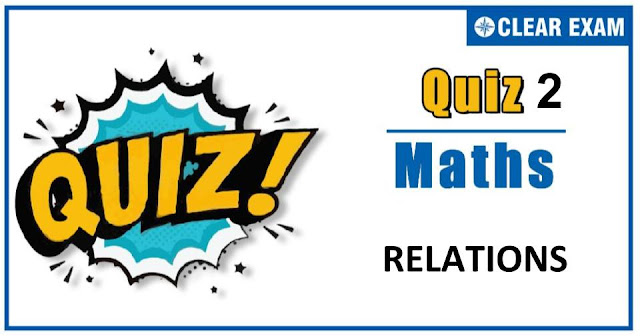## Dear Readers,As per analysis for previous years, it has been observed that students preparing for JEE MAINS find Mathematics out of all the sections to be complex to handle and the majority of them are not able to comprehend the reason behind it. This problem arises especially because these aspirants appearing for the examination are more inclined to have a keen interest in Mathematics due to their ENGINEERING background. Furthermore, sections such as Mathematics are dominantly based on theories, laws, numerical in comparison to a section of Engineering which is more of fact-based, Physics, and includes substantial explanations. By using the table given below, you easily and directly access to the topics and respective links of MCQs. Moreover, to make learning smooth and efficient, all the questions come with their supportive solutions to make utilization of time even more productive. Students will be covered for all their studies as the topics are available from basics to even the most advanced.

Q2. If f(x)=sin2⁡(x,g(x)=√x) and h(x)=cos-1⁡(x,0≤x≤1,) then
•  hogof=fogoh
•  gofoh=fohog
•  fohog=hogof
•  None of these
Solution
We have,
hogof (x)=cos-1⁡(|sin⁡x | )
and,fogoh (x)=sin2⁡(√(cos-1⁡x ))
Clearly, hogof (x)≠fogoh(c)
Thus, option (a) is not correct
Now, gofoh (x)=|sin⁡(cos-1⁡x ) |=|sin⁡(sin-1⁡√(1-x2 ) ) |=√(1-x2 )

Q3.  If f(x) and g(x) are two real functions such that f(x)+g(x)=ex and f(x)-g(x)=e-x, then
•  f(x) is an odd function
•  g(x) is an even function
•  f(x) and g(x) are periodic functions
•  None of these
Solution
Q6. If f(x) is an odd function, then the curve y=f(x) is symmetric

Solution
Q9.
•  Even
•  Odd
•  Neither even nor odd
•  Periodic with period π

Q10. The number of reflexive relations of a set with four elements is equal to
•  216
•  212
•  28
•  24
Solution
Number of reflexive relations of a set of 4 elements=2(42)-4 =212## Want to know more

Want to Know More
Please fill in the details below:

## Latest NEET Articles\$type=three\$c=3\$author=hide\$comment=hide\$rm=hide\$date=hide\$snippet=hide

Name

ltr
item
Best IIT JEE Coaching Institute in Delhi | NEET Coaching Institute in Delhi: RELATIONS-QUIZ-2
RELATIONS-QUIZ-2
https://1.bp.blogspot.com/-F0kDdSHL4mM/X31qAk5xyeI/AAAAAAAABBA/3ewwv4R2EjYlCYjOm49W9WXIwIHYN59bgCLcBGAsYHQ/w640-h336/Quiz%2BImage%2BTemplate%2B%25289%2529.jpg
https://1.bp.blogspot.com/-F0kDdSHL4mM/X31qAk5xyeI/AAAAAAAABBA/3ewwv4R2EjYlCYjOm49W9WXIwIHYN59bgCLcBGAsYHQ/s72-w640-c-h336/Quiz%2BImage%2BTemplate%2B%25289%2529.jpg
Best IIT JEE Coaching Institute in Delhi | NEET Coaching Institute in Delhi
https://www.cleariitmedical.com/2020/10/RELATIONS-QUIZ-2.html
https://www.cleariitmedical.com/
https://www.cleariitmedical.com/
https://www.cleariitmedical.com/2020/10/RELATIONS-QUIZ-2.html
true
7783647550433378923
UTF-8